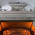## Math Geeking out

So I came across this really cool anomoly in simple arithmatic.

Pick any number that is a non-multiple of 7.

Now divide it by 7.

I don't know what the answer is to your specific number, but I can tell you one thing for certain. It's a number that repeats these six digits forever: "142857"

Crazy, right?

Go ahead give it a try:

1/7= _____
2/7= _____
3/7= _____
4/7= _____
5/7= _____
6/7= _____
8/7= _____
6,000/7= _____

WILD!

Also, I finally have a new video coming out next Wednesday, so keep an eye out for it.

1.having become a pseudo math theorist i recognized the pattern you have up somewhat quickly. i don't know all of the intricacies but it is related to the 22/7 = pi phenomena. before modern advancements in mathematics 22/7 was the standard for pi, as a result the pattern you recognized in dividing non multiples by 7 is their relation to the curve of a perfect circle. like you said, a completely irrational number but a measurable occurance! fun with numbers!

2.In my experiments (mostly test 1) I have found that this is not always the case.

(9/7)/9 is not equal to (6/7)/6 and some other cases (marked red). I find no pattern to when, or why, this occurs.

But I don't do the same math as you do in your post.

3.The fact that you’re thinking about this for fun, somehow reminds me of the Barbie doll that says, “Math class is tough.”

4.That's interesting, but wait a minute...a repeating decimal is not an irrational number. If you dividing an integer by any other integer, in this case 7, by definition you get a rational number. You may get a repeating decimal if you choose to represent the answer as a decimal, but n/7 is a rational number for any rational number n. Irrational numbers by definition cannot be expressed as a ratio of two integers.

5.Thanks Bob for catching my slip up!